# JASP for Bayesian analyses with default priors

Welcome!

This tutorial illustrates how to perform Bayesian analyses in JASP (JASP Team, 2020) with default priors for beginners. We deal with basic procedures to do Bayesian statistics and explain ways to interpret core results.

In each analytic option, a brief comparison between Bayesian and frequentist statistics is presented. After the tutorial, we expect readers can perform correlation analysis, multiple linear regression, t-test, and one-way analysis of variance, all from a Bayesian perspective, and understand the logic of Bayesian statistics.

For readers who need a comprehensive understanding of what the JASP is, how to install it, how to manage and explore data, and how to interpret the statistical analyses from a frequentist perspective, we recommend reading JASP for beginners.

Since we continuously improve the tutorials, let us know if you discover mistakes, or if you have additional resources we can refer to. If you want to be the first to be informed about updates, follow Rens on Twitter.

Let’s get started!

### How to cite this tutorial in APA style

Heo, I., Veen, D., & Van de Schoot, R. (2020, July 16). JASP for Bayesian analyses with default priors. Zenodo. https://doi.org/10.5281/zenodo.4008339

## Popularity Dataset

We will use a popularity dataset (Van de Schoot, 2020) from Van de Schoot, Van der Velden, Boom, and Brugman (2010). To download the dataset, click here.

The dataset is based on a study that investigates an association between popularity status and antisocial behavior from at-risk adolescents (n = 1491), where gender and ethnic background are moderators under the association. The study distinguished subgroups within the popular status group in terms of overt and covert antisocial behavior.

For more information on the sample, instruments, methodology, and research context, we refer the interested readers to the paper (see references). A brief description of the variables in the dataset follows. The variable names in the table below will be used in the tutorial, henceforth.

Variable name Description
respnr Respondents’ number
Dutch Respondents’ ethnic background (0 = Dutch origin, 1 = non-Dutch origin)
gender Respondents’ gender (0 = boys, 1 = girls)
covert Covert antisocial behavior
overt Overt antisocial behavior

### How to cite the dataset in APA style

Van de Schoot, R. (2020). Popularity Dataset for Online Stats Training [Data set]. Zenodo. https://doi.org/10.5281/zenodo.3962123

## I. We go together with data

• Statistical analysis always goes along with data.
• To download the dataset that we will use (popular_regr_1), click here.
• With several mouse clicks, we can easily load the data. JASP offers three ways of loading data: from your computer, the in-built data library, or the Open Science Framework.

## II. Another ‘earth’: Bayesian statistics

• Bayesian statistics and frequentist statistics are two different branches in the world of statistics. Their distinctive approaches lead to different ways of statistical inference. Thus, they live on their respective earth.
• In Bayesian statistics, the prior distributions are combined with the likelihood of data to update the prior distributions to the posterior distributions. The updated posterior distributions about parameters of interest are used for statistical inference. This logic of Bayesian statistics implies that how the prior distributions are specified plays a crucial role in doing Bayesian statistics.
• In this tutorial, we rely on the default prior settings in JASP.
• For a more concrete explanation about Bayesian statistics, we refer interested readers to the two great easily-written papers.

## III. Time to perform Bayesian analyses

• We assume that all the assumptions required for subsequent analyses are satisfied.
• In each analysis, we start by providing a brief comparison between the frequentist and the Bayesian approach for the chosen analysis. The procedures to perform the Bayesian analysis follows the comparison. Then, you encounter the interpretation of results.

### 1. Correlation analysis

• When you are interested in investigating the strength of the relationship between two variables, correlation analysis can be an option.
• In frequentist statistics, the focus is to examine whether the correlation coefficient is significantly different from 0 or not. The significance level is usually set to .05. If a p-value is lower than the significance level, the null hypothesis that the correlation coefficient between two variables is 0 is rejected. The alternative hypothesis is that the correlation coefficient is not equal to 0.
• In Bayesian statistics, the null hypothesis postulates that the correlation coefficient between the two variables is 0. In other words, two variables are not correlated. The alternative hypothesis, however, states that the correlation coefficient exists. That is, two variables are correlated.
• Between the null and the alternative hypothesis, Bayesian statistics quantifies the relative support toward each hypothesis from observed data. The Bayes factor shows this quantification. In JASP, the default Bayes factor is $$BF_{10}$$. This value means how much the observed data relatively supports the alternative hypothesis ($$H_1$$) than the null hypothesis ($$H_0$$).
• For a better grasp, let’s examine whether two variables in the dataset, the covert and overt antisocial behavior (covert and overt) are correlated.
• Go to the top bar -> Click Regression -> Click Bayesian Correlation under Bayesian section
• Foremost, we will set a seed to guarantee that results are reproducible. Any numbers are possible. This tutorial uses a seed of 123.
• Click Options in the control panel -> Check Set seed under Repeatability -> Type 123
• In our example, move covert and overt under the Variables section. Next, check Report Bayes factors and Credible intervals under Additional Options in the control panel.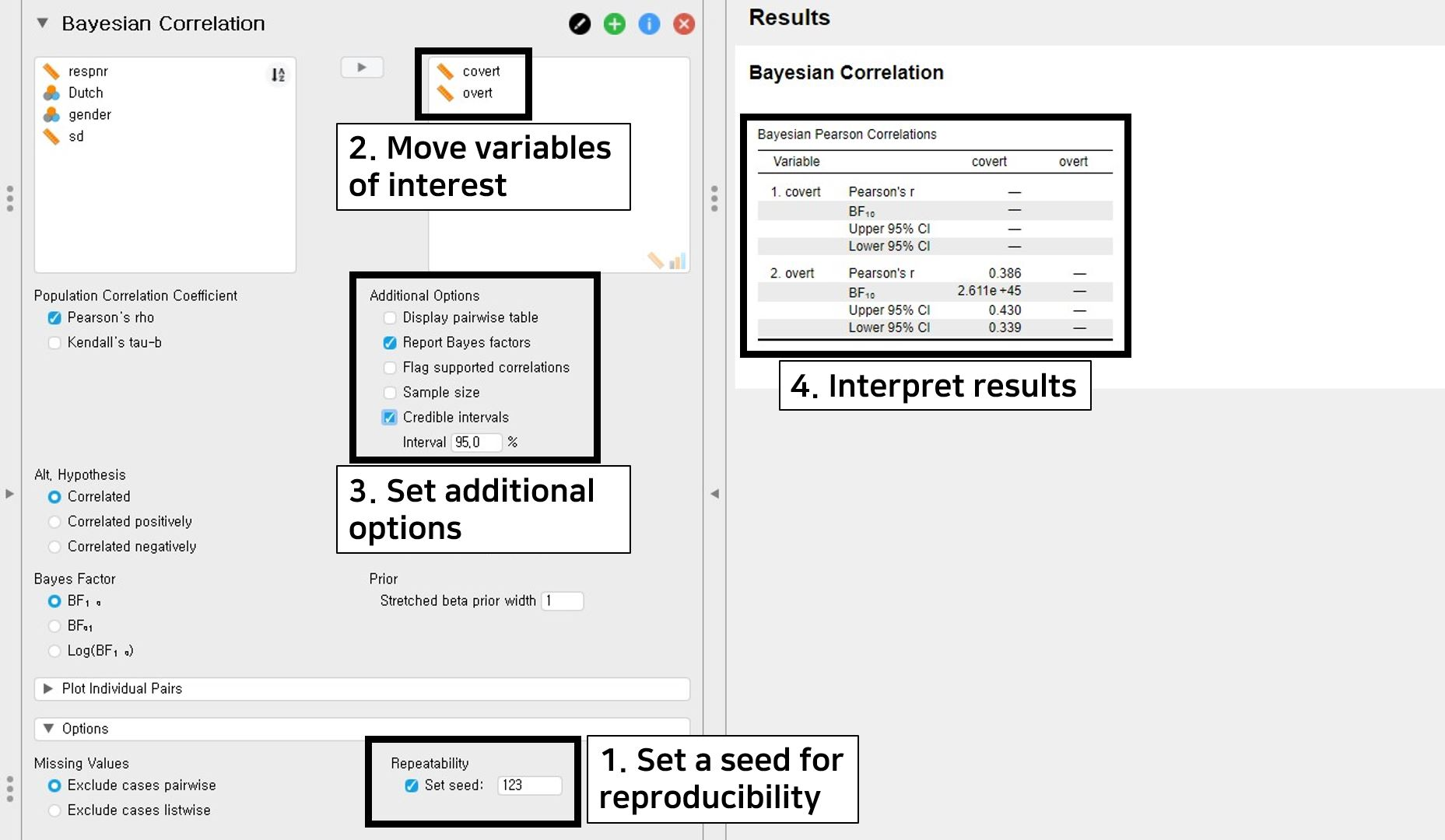• The correlation coefficient between covert and overt is 0.386 and the $$BF_{10}$$ is 2.611e+45. The number 2.611e+45 equals $$2.611 × 10^{45}$$. This means that the support in the observed data is about $$2.611 × 10^{45}$$ times larger for the alternative hypothesis (i.e., covert and overt are correlated) than for the null hypothesis (i.e., covert and overt are not correlated).
• The upper and lower limit of 95% credible interval is 0.430 and 0.339. We can check that 0 is not included in the 95% credible interval. It means that there is a 95% probability that the correlation coefficient between covert and overt lies in the population within the corresponding interval. Thus, it is fairly sure that covert and overt are correlated.
• Notice that the Bayesian statistics is not concerned with rejecting or not rejecting the null hypothesis. Rather, it is keen on how much the observed data supports the alternative or the null hypothesis.

### 2. Multiple linear regression

• Multiple linear regression analysis is performed when you are interested in predicting outcomes of a continuous dependent variable with multiple continuous or categorical independent variables.
• In the frequentist framework, we examine whether the regression coefficient is significantly different from 0. If the p-value is lower than the significance level, the null hypothesis that the regression coefficient is 0 is rejected. The alternative hypothesis is that the regression coefficient is not equal to 0.
• In the Bayesian framework, the null hypothesis states that the independent variables do not predict the dependent variables. The alternative hypothesis states that there exist effects of independent variables in predicting the dependent variables.
• For illustrative purposes, let’s try to predict adolescents’ socially desirable answering patterns (sd) with overt and covert antisocial behavior (overt and covert). Thus, we use sd as the dependent variable and covert and overt as the independent variables.
• Go to the top bar -> Click Regression -> Click Linear Regression under Bayesian section
• Let’s first set a seed of 123 for replicable results.
• Click Advanced Options in the control panel -> Check Set seed under Repeatability -> Type 123
• For our purposes, move sd under the Dependent Variable section and covert and overt under the Covariates section. Check the Posterior summary under Output in the control panel. Instead of the Model averaged option, we choose the Best model option to see the estimates under the most probable model given the observed data.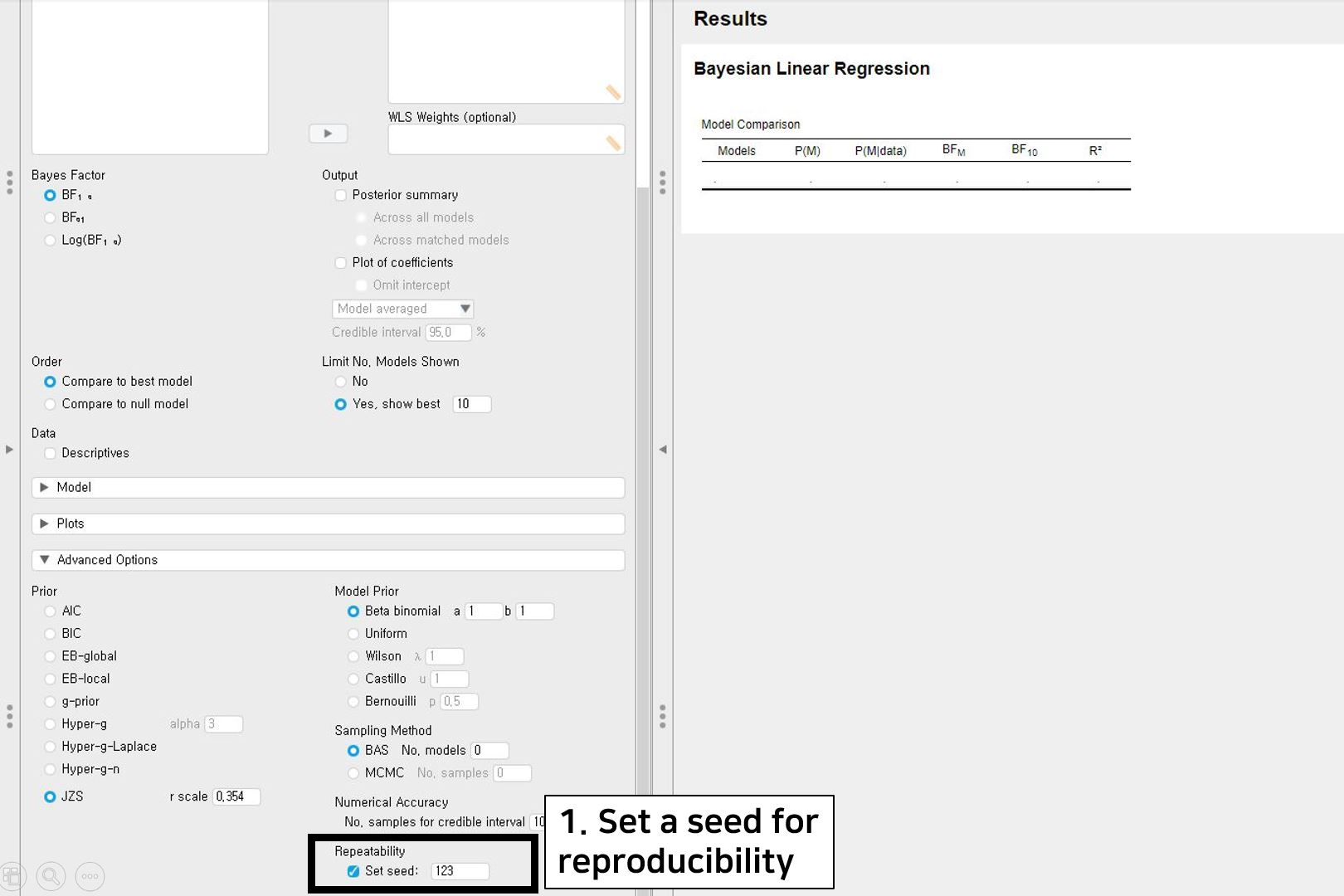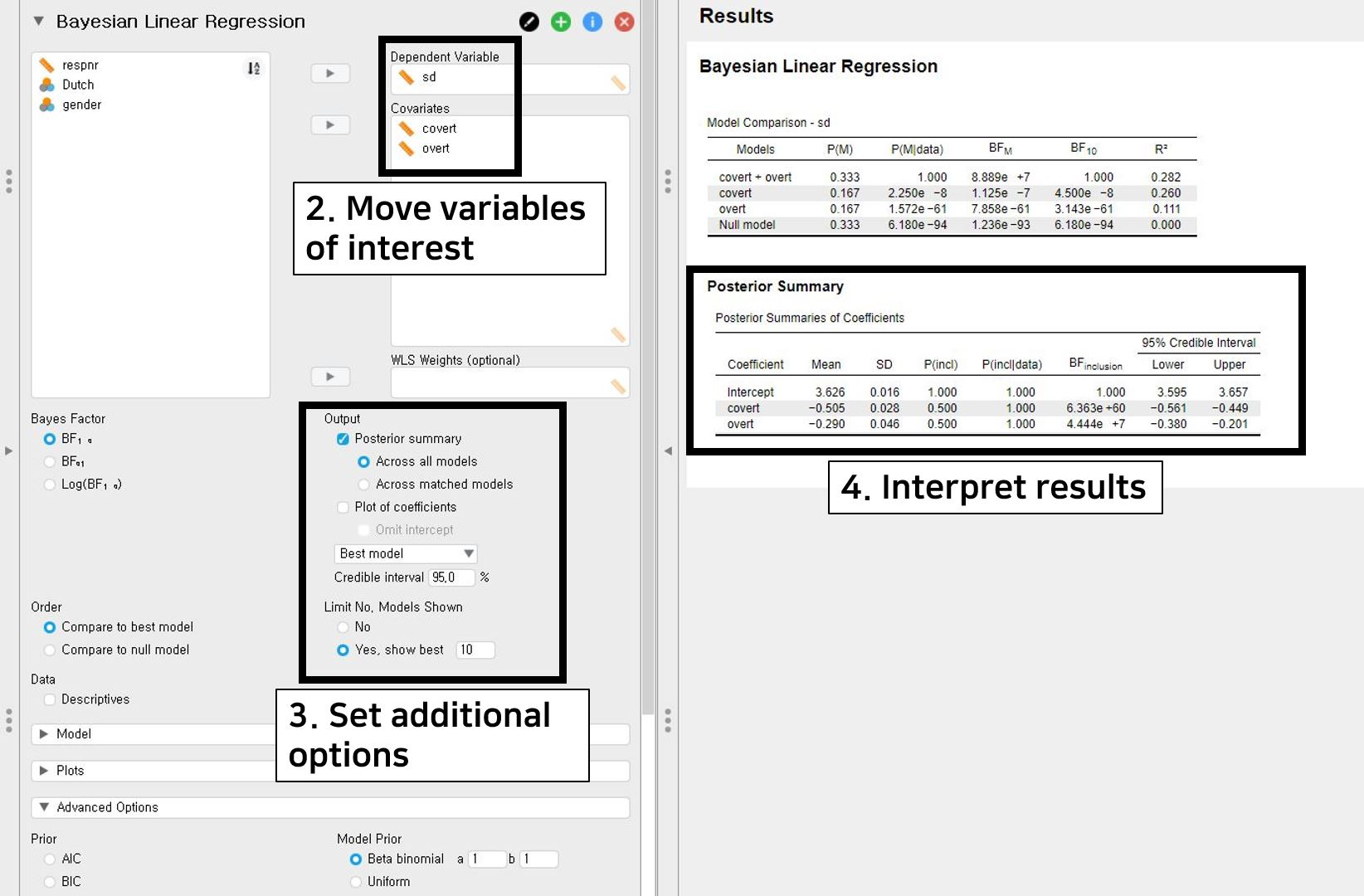• To interpret the results, look at the table under Posterior Summary in the output panel. As the title ‘Posterior Summary’ says, statistical inferences in Bayesian statistics are focused on the posterior distribution. The posterior summary shows the summary of regression coefficients after taking into account the default prior and the likelihood of data. The Mean and the SD refer to the posterior mean and the posterior standard deviation.
• The posterior mean of the regression coefficient of covert is -0.505. We can interpret this estimate such that one unit increase in the covert leads to a decrease of 0.505 unit in the sd on average. The 95% credible interval of [-0.561, -0.449] indicates that there is a 95% probability that the regression coefficient of covert lies in the population within the corresponding credible interval. Also, the 95% credible interval does not include 0, so it is fairly sure that there is an effect of covert in predicting sd.
• The posterior mean of the regression coefficient of overt, on the other hand, is -0.290. This is interpreted such that one unit increase in overt leads to a decrease of 0.290 in sd on average. According to the 95% credible interval of [-0.380, -0.201], we are 95% sure that the regression coefficient of overt lies within the corresponding interval in the population. Furthermore, 0 is not included in the 95% credible interval. Thus, there is fair evidence that overt predicts sd.

### 3. t-test

• If you are interested in investigating whether the means of certain variables between the two groups are different from each other, a t-test can be an option.
• From a frequentist perspective, the focus is to investigate whether the means between the two groups are significantly different. If the p-value is lower than the significance level, the null hypothesis that the mean difference between the two groups is equal to 0 is rejected. The alternative hypothesis postulates that the mean difference is significantly different from 0.
• From a Bayesian perspective, the null hypothesis states that there is no mean difference between the two groups. The alternative hypothesis, on the other hand, is that there is a mean difference between the two groups.
• Let’s examine whether the means of covert antisocial behavior (covert) between the respondents’ different ethnic backgrounds (Dutch) are different.
• Go to the top bar -> Click T-Tests -> Click Independent Samples T-Test under Bayesian section
• Because we assumed that all assumptions are met, we will use the Student t-test. For the Student t-test, we do not need to set seed! Thus, we go directly to the next step.
• If you use the Mann-Whitney U test since the assumptions of the independent samples t-test are violated, it is possible to set seed to have a replicable output.
• Move the covert variable under the Variables section and the Dutch variable under the Grouping Variable section.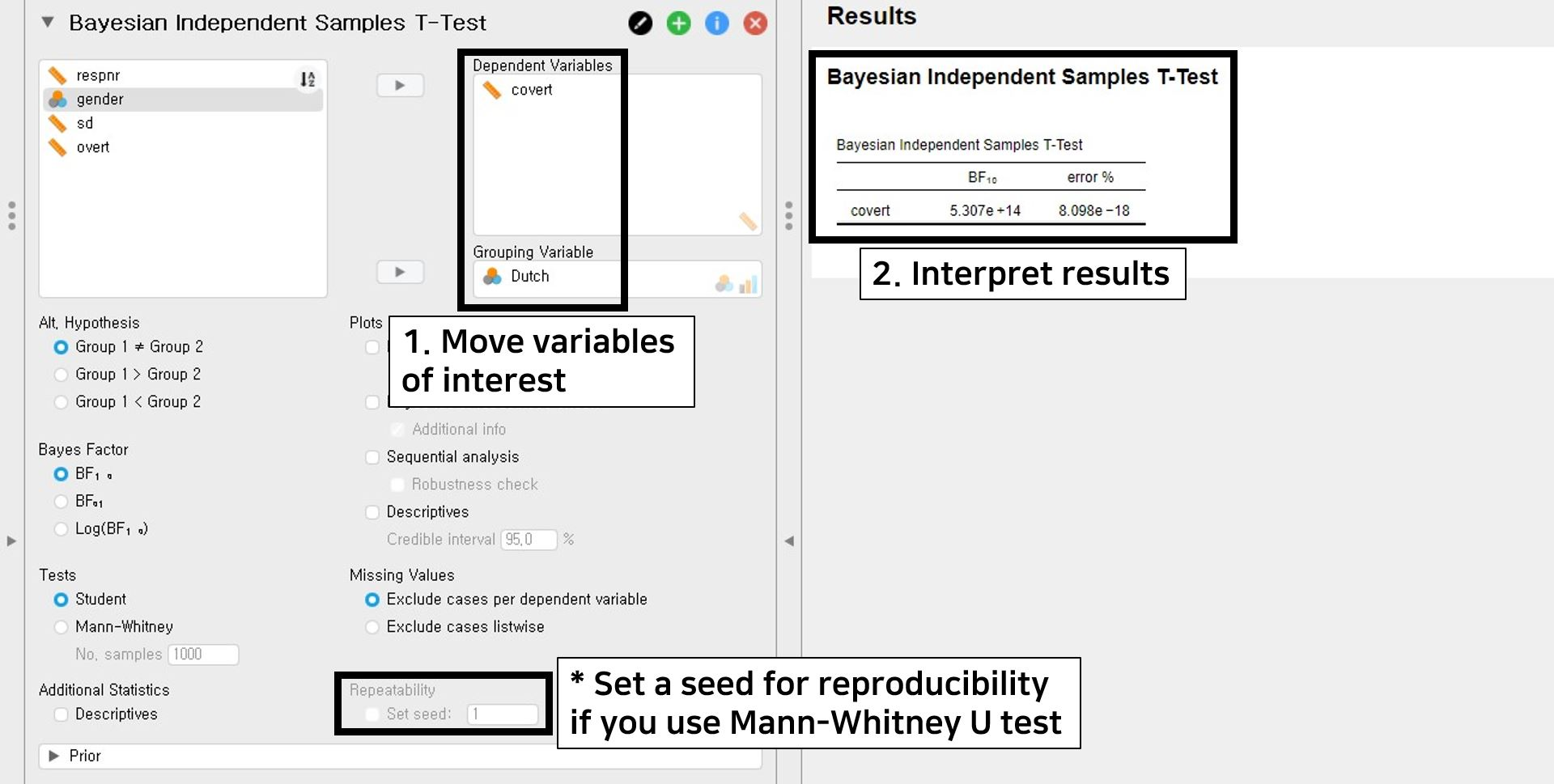• The table under the Bayesian Independent Samples T-Test provides the output. The number 5.307e+14 of $$BF_{10}$$ is equal to $$5.307 × 10^{14}$$. This means that the observed data support $$5.307 × 10^{14}$$ times more for the alternative hypothesis (i.e., there is a mean difference of covert between the two groups of the Dutch variable) than for the null hypothesis (i.e., A mean difference of covert between the two groups of the Dutch variable does not exist).
• The error % (error percentage) is 8.098e-18, which is $$8.098 × 10^{-18}$$. Because the error percentage is low enough, it indicates that the numerical results are robust.

### 4. One-way analysis of variance

• When you want to examine whether the means are different across groups, one-way analysis of variance answers your question.
• In the frequentist statistics, we aim to examine whether the means across different groups are significantly different from each other. If the p-value is lower than the prespecified significance level, the null hypothesis that there is no mean difference across groups is rejected. The alternative hypothesis states that there are significant mean differences across groups.
• In the Bayesian statistics, the null hypothesis says that there is no mean difference across different groups. However, the alternative hypothesis postulates that there exist mean differences across different groups.
• As an example, we will examine whether the differences in the mean of covert antisocial behavior (covert) exist across respondents’ genders (gender).
• Before going further, there is one thing we should do. In the dataset, the gender variable has three categories: 0, 1, and 99. Since JASP treats 99 as an independent category, which is not the case, it is necessary to filter 99 out. We expect you are familiar with filtering.
• If not, please go to JASP for beginners and see how to filter a certain category of the variable out.
• We proceed with the next step assuming that you filtered the 99 category of the gender variable out!
• Go to the top bar -> Click ANOVA -> Click ANOVA under Bayesian section -> Choose one dependent variable for Dependent Variable and grouping variables for Fixed Factors
• To have repeatable results, we will set a seed of 123.
• Click Advanced Options in the control panel -> Check Set seed under Repeatability -> Type 123
• Move the covert variable under the Dependent Variable section and the gender variable under the Fixed Factors section. Next, check Compare to null model under Order in the control panel.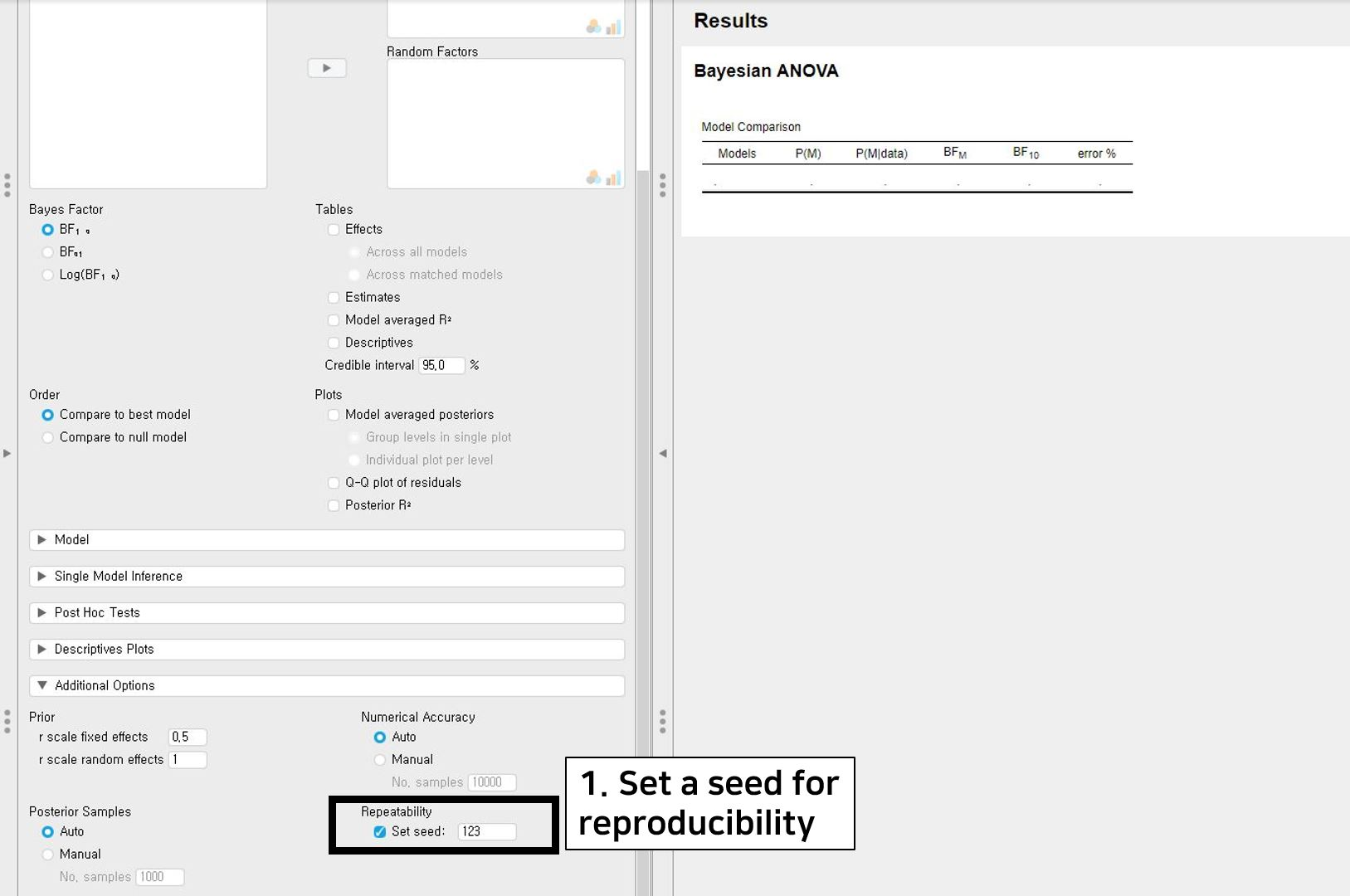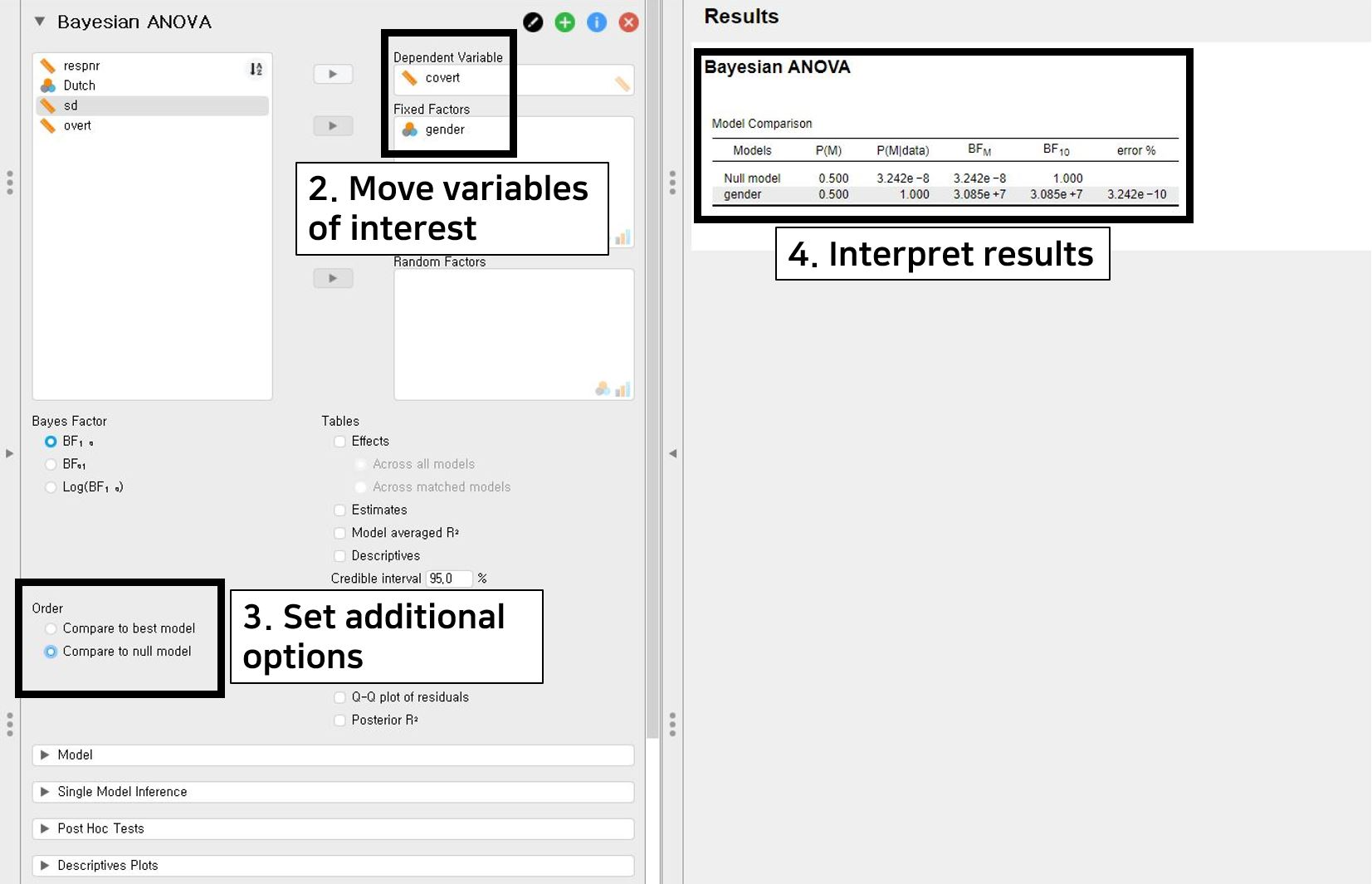• Let’s take a look at the table under the Bayesian ANOVA in the output panel. The Null model only contains the grand mean. The gender model (denoted as gender in the first column) adds an effect of the gender variable to the null model. Consequently, we have to look at the ‘gender’ model row.
• The value 3.085e+7 of $$BF_{10}$$ equals $$3.085 × 10^{7}$$. This implies that the support is $$3.085 × 10^{7}$$ times larger for the alternative hypothesis (i.e., there exists mean differences of covert across gender groups) than for the null hypothesis (i.e., mean differences of covert do not exist across gender groups).
• As explained in the t-test part, the error percentage indicates the numerical robustness of results. The error percentage of 3.242e-10 is equal to $$3.242 × 10^{-10}$$. This value is lower enough to show that the results are stable.

## IV. Epilogue

• Congratulations! You have reached the finish line of this tutorial. We hope you enjoyed it.
• This tutorial dealt with simple but core steps excluding other advanced options. We want to emphasize, however, that there are more interesting concepts to explain in doing Bayesian analyses.
• We thus recommend following the tutorial Advanced Bayesian regression in JASP. It provides an in-depth explanation for an advanced understanding of outputs and other options in performing Bayesian regression analysis.
• It should be noted that we performed the Bayesian analyses with the default priors. Of course, it is possible to use the informative priors depending on, for instance, previous literature or expert knowledge.
• For readers interested in the effects of other priors specifications, we suggest following the tutorial WAMBS Checklist in JASP (using Jags). It additionally explains how to sensibly apply Bayesian analyses for substantive research questions.